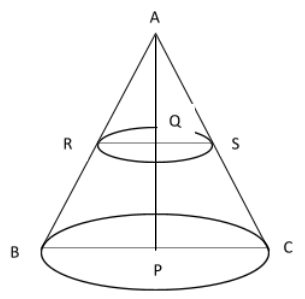Courses
Courses for Kids
Free study material
Offline Centres
MoreLast updated date: 27th Nov 2023
Total views: 280.8k
Views today: 5.80k

# A metallic right circular cone $20cm$ high and whose vertical angle is ${60^ \circ }$ is cut into two parts at the middle of its height by a plane parallel to its base. If the frustum is drawn into a wire of diameter $\dfrac{1}{{16}}cm$, find the length of the wire.Verified
280.8k+ views
Hint: In this question they have asked to find the length of the wire used to draw the frustum which is formed when a plane parallel to the right circular cone cuts the cone. So we will first find the volume of the frustum formed, then we will calculate the volume of a cylindrical wire with diameter $\dfrac{1}{{16}}cm$. Equate the obtained two volumes to get the required length of the wire.

Complete step-by-step solution:
Consider the following figure drawn according to the data given, let $$BCRS$$ be the metallic right circular cone of height, $AP = 20cm$ also given that the vertical angle $$\angle BAC = {60^ \circ }$$, since $AP$ is a bisector we get $\angle PAC = {30^ \circ } = \angle QAS$. Since the cone is cut in the middle we have $AQ = 10cm = QP$$BC$ is the base of the cone which is cut by the parallel plane $$RS$$ to form the frustum $BCRS$. We have to find the length of the wire needed to draw the frustum. Let the length of wire be $h$.
Since frustum is drawn into wire we can find the length of wire using the relation,
Volume of frustum $$BCRS$$= Volume of the cylindrical wire with diameter $\dfrac{1}{{16}}cm$.
First let us find the volume of frustum $$BCRS$$. The formula for volume of frustum is given by,
${V_1} = \dfrac{{\pi h}}{3}({r_1}^2 + r_2^2 + {r_1}{r_2})$ , here height of the cone $h = 20cm$ , ${r_1}$ is the radius of base $$BC$$ and ${r_2}$ is the radius of the smaller base $$RS$$.
From the figure ${r_1} = PC$ and ${r_2} = QS$, now we will find ${r_1}$ and ${r_2}$ using the right angle triangles $\blacktriangle APC$ and $\blacktriangle AQS$ respectively ,
In $\blacktriangle APC$, we can write
$\tan (\angle PAC) = \dfrac{{opposite}}{{adjacent}} = \dfrac{{PC}}{{AP}}$
$\Rightarrow \tan {30^ \circ } = \dfrac{{{r_1}}}{{20}}$
$\Rightarrow \dfrac{1}{{\sqrt 3 }} = \dfrac{{{r_1}}}{{20}}$
$\Rightarrow {r_1} = \dfrac{{20}}{{\sqrt 3 }}cm$
From $\blacktriangle AQS$ we will find the value of ${r_2}$ in the same manner , i.e.
$\tan (\angle QAS) = \dfrac{{opposite}}{{adjacent}} = \dfrac{{QS}}{{AQ}}$
$\Rightarrow \tan {30^ \circ } = \dfrac{{{r_2}}}{{10}}$
$\Rightarrow \dfrac{1}{{\sqrt 3 }} = \dfrac{{{r_2}}}{{10}}$
$\Rightarrow {r_2} = \dfrac{{10}}{{\sqrt 3 }}cm$
Substituting these values in the formula for volume of frustum $$BCRS$$ we have,
\eqalign{ & {V_1} = \dfrac{{\pi h}}{3}({r_1}^2 + r_2^2 + {r_1}{r_2}) = \dfrac{{10\pi }}{3}\left\{ {{{\left( {\dfrac{{20}}{{\sqrt 3 }}} \right)}^2} + {{\left( {\dfrac{{10}}{{\sqrt 3 }}} \right)}^2} + \dfrac{{20}}{{\sqrt 3 }} * \dfrac{{10}}{{\sqrt 3 }})} \right\} \cr & = \dfrac{{10\pi }}{3}\left\{ {\dfrac{{400}}{3} + \dfrac{{100}}{3} + \dfrac{{200}}{3}} \right\} = \dfrac{{10\pi }}{3} \times \dfrac{{700}}{3} = \dfrac{{7000\pi }}{9}c{m^3} \cr}
Now to find the volume of cylindrical wire of diameter $\dfrac{1}{{16}}cm$, first we will find the radius which is half of the diameter , i.e. $$r = \dfrac{{\left( {\dfrac{1}{{16}}} \right)}}{2} = \dfrac{1}{{16 \times 2}} = \dfrac{1}{{32}}cm$$
Volume of cylindrical wire, ${V_2} = \pi {r^2}h = \pi {\left( {\dfrac{1}{{32}}} \right)^2}h$
Now equating both the volumes we get the value of $h$,
\eqalign{ & \dfrac{{7000\pi }}{9} = \dfrac{{\pi h}}{{32 \times 32}} \cr & \Rightarrow h = \dfrac{{7000\pi \times 32 \times 32}}{{9\pi }} = 796444.44cm = 7964.44m \cr}
Therefore the length of the wire required is $7964.44m$.

Note: Right circular cone is a cone in which the center point of the circular base is joined with the apex of the cone which forms the right angle with respect to the base and it bisects the vertical angle at the apex. When a cone is cut by a plane parallel to the base it divides the cone into two parts, the upper part of the cone remains the same in shape whereas the bottom part makes the frustum .